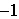Name:    Math 9 1.1 Squares and square roots

Multiple Choice
Identify the choice that best completes the statement or answers the question.

1.

How many of the following are perfect squares?
16, 24, 36, and 45
 a. One c. Three b. Two d. None

2.

Simplify each of the following square roots: (NO Calculators)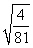a.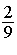c.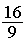b.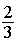d.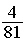3.

Simplify each of the following square roots: (NO Calculators)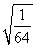a.c.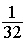b.d.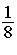4.

Simplify each of the following square roots: (NO Calculators)a.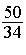c.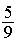b.d.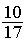5.

Simplify each of the following square roots: (NO Calculators)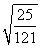a.c.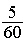b.d.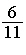6.

Simplify each of the following square roots: (NO Calculators)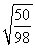a.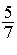c.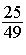b.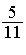d.7.

Simplify each of the following square roots: (NO Calculators)a.c.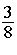b.d.8.

Simplify each of the following square roots: (NO Calculators)a.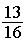c.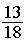b.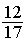d.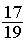9.

Find the value of the unknown: (No calculators)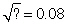a.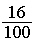c.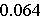b.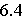d.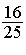10.

If the area of a square is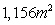, what is the length of its side?
 a. 15m c. 34m b. 578m d. 289m

11.

Find the side length of the following square: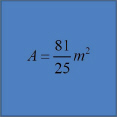a. 1.2m c. 1.6m b. 1.4m d. 1.8m

12.

Find the side length of the following square:a.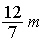c. 1.54m b.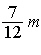d. 1.42

13.

Find the side length of the following square: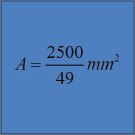a.c.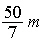b.d.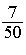m

Multiple Response
Identify one or more choices that best complete the statement or answer the question.

14.

Given all the numbers below, check all the ones that are pefect squares
 a. 81 c. 71 e. 169 b. 225 d. 144 f. 1000

15.

Given all the numbers below, check all the perfect squares:
 a. 25 c. 0 e. 8 b. 125 d. 1 f.